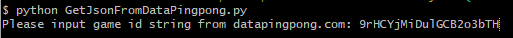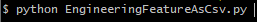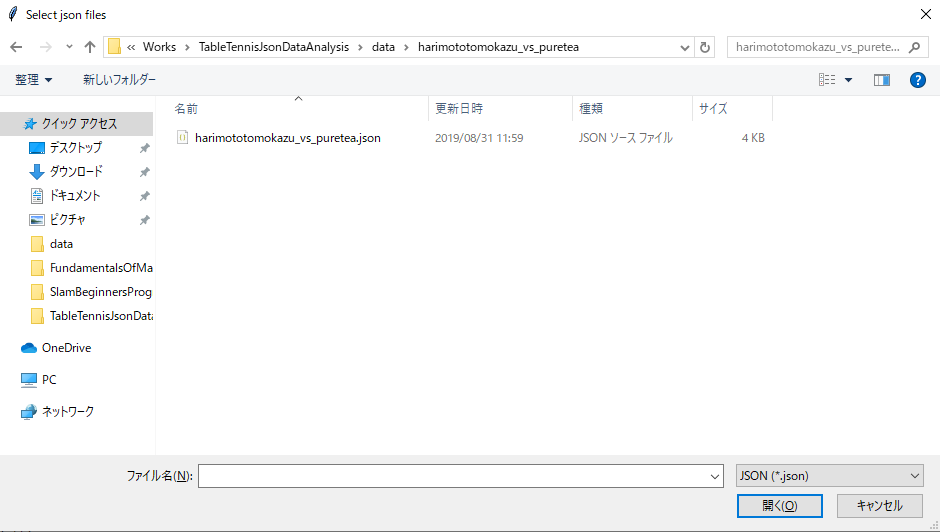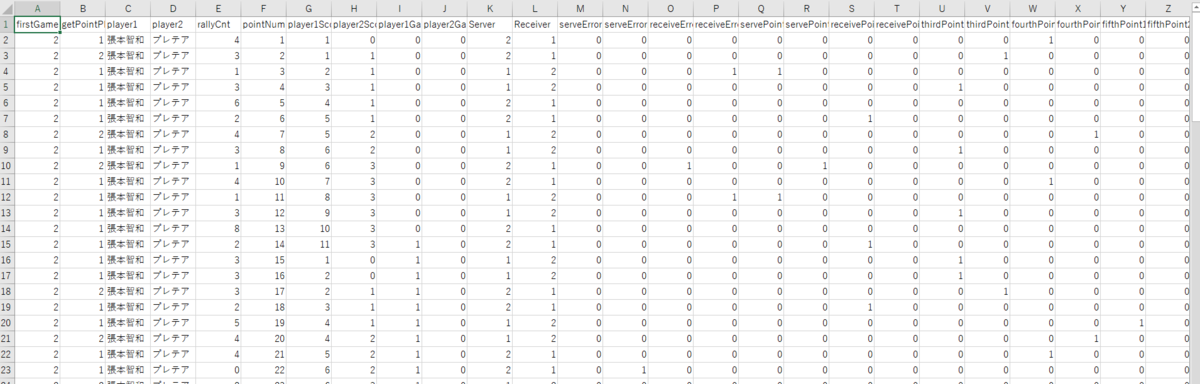# Momentum shift analysis of Table tennis game ~Data collection~

## Introduction

I have been playing table tennis since I was a junior high school student and it is my favorite sport. I am interested in not only playing but also analyzing data. So, I will start collecting data of table tennis games and analyzing it by myself. In this article, I am introducing how to collect data and calculating additional feature values.

## Analysis target

There is a momentum shift at almost sports games. About table tennis, when one side player is leading by a lot of points, the other player sometimes come from behind to win. The momentum shift like this is invisible directly. I think that there is a different thing between a come from behind win and a come from ahead loss. I will try analyzing the difference from now on.

## Prior knowledge

• Table tennis game is 5 or 7 games match.
• Each game is 11 points match.
• Each player serves 2 of each alternatively.

## How to collect data

I will collect data from the following website "datapingpong".

A manager of this site, Mr. Genki Ishikawa released an api to get saved scoring data of a game. I can get the data as JSON file. How to use the api and the contents of data in detail is explained at the following article.

### Python script

I created a Python script to get a released data as JSON file with the above api. The source code of script is as follow.

```# -*- coding: utf-8 -*-

import requests
import json
from pykakasi import kakasi

game_id = input('Please input game id string from datapingpong.com: ')

game_data = requests.get("https://us-central1-datapingpong-vue.cloudfunctions.net/gameData?id={}".format(game_id))

game_data.encoding = game_data.apparent_encoding

game_data_json = game_data.json()

player1_jpn = game_data_json['player1']
player2_jpn = game_data_json['player2']

kakasi = kakasi()
kakasi.setMode("H", "a")
kakasi.setMode("K", "a")
kakasi.setMode("J", "a")
kakasi.setMode("r", "Hepburn")
conv = kakasi.getConverter()
player1_ascii = conv.do(player1_jpn)
player2_ascii = conv.do(player2_jpn)

save_file_name = player1_ascii + '_vs_' + player2_ascii + '.json'

with open(save_file_name, 'w') as f:
json.dump(game_data_json, f, ensure_ascii=False, indent=4)
```

### Usage

For example, you can get the data by executing the following command.
After this command was executed, you will be asked to input game ID.The data will be saved as JSON file at the same directly where the script is located.

Based on some original data in the above JSON file, I calculated the following 32 kinds data as feature values for my analysis.

### Feature values

• Server of first game
• Player who get point
• Name of player 1/2
• Rally count
• Point number
• Point count of player 1/2
• Game count of player 1/2
• Serve error flag of player 1/2
• Receive error flag of player 1/2
• Serve point flag of player 1/2
• Receive point flag of player 1/2
• Third point flag of player 1/2
• Fourth point flag of player 1/2
• Fifth point flag of player 1/2
• Sixth point flag of player 1/2
• Long rally point flag of player 1/2(Rally count is over than 7)
• Consecutive point count of player 1/2

### Python script

I created a Python script to calculate these feature values and save them as CSV file. The source code of script is as follow.

```# -*- coding: utf-8 -*-

import tkinter as tk
import tkinter.filedialog as tkfd
import pandas as pd
import numpy as np
import json

class EngineeringFeatureAsCsv:
def __init__(self):
self.json_path_list     = None
self.json_data          = None
self.player_1_name      = None
self.player_2_name      = None
self.server_num         = None
self.first_server_num   = None
self.sum_score_1        = 0
self.sum_score_2        = 0
self.sum_game_count_1   = 0
self.sum_game_count_2   = 0
self.consec_count_1     = 0
self.consec_count_2     = 0
self.df_data            = None

fType = [('JSON', '*.json')]
filetypes=fType)
if not self.json_path_list:
print('Select json files')
else:
for path in self.json_path_list:
with open(path, 'r') as f:
self.get_first_server_num()
self.get_player_name()
self.convert_json_to_df(path)
self.create_features()
self.save_as_csv(path)

def get_player_name(self):
if self.json_data:
self.player_1_name = self.json_data['player1']
self.player_2_name = self.json_data['player2']

def get_first_server_num(self):
if self.json_data:
self.server_num = self.json_data['firstGameServer']
self.first_server_num = self.server_num
if self.server_num == 1:
else:

def convert_json_to_df(self, path):
df_drop_memo  = df_org.drop('memo', axis=1)
df_drop_match = df_drop_memo.drop('matchName', axis=1)
self.df_data  = df_drop_match

def save_as_csv(self, path):
data_name = (path.split('/')[-1]).split('.')
save_name = data_name + '.csv'
self.df_data.to_csv(save_name, index=False, encoding='shift-jis')

self.server_array[index]   = self.server_num
if (self.sum_score_1 + self.sum_score_2) % 2 == 0:
if self.server_num == 1:
self.server_num   = 2
else:
self.server_num   = 1
if self.sum_score_1 == 0 and self.sum_score_2 == 0:
if self.first_server_num == 1:
self.server_num = 2
self.first_server_num = self.server_num
else:
self.server_num = 1
self.first_server_num = self.server_num

def count_game(self, index):
if self.sum_score_1 > self.sum_score_2:
self.sum_game_count_1 += 1
else:
self.sum_game_count_2 += 1
self.game_count_1_array[index] = self.sum_game_count_1
self.game_count_2_array[index] = self.sum_game_count_2

def set_serve_error(self, index, gpp, rc):
if rc == 0:
if gpp == 1:
self.serve_error_2_array[index] = True
else:
self.serve_error_1_array[index] = True

if rc == 1:
if gpp == 1:
self.serve_point_1_array[index]   = True
else:
self.serve_point_2_array[index]   = True

if rc == 2:
if gpp == 1:
else:

def set_third_point(self, index, gpp, rc):
if rc == 3:
if gpp == 1:
self.third_point_1_array[index]   = True
else:
self.third_point_2_array[index]   = True

def set_fourth_point(self, index, gpp, rc):
if rc == 4:
if gpp == 1:
self.fourth_point_1_array[index]   = True
else:
self.fourth_point_2_array[index]   = True

def set_fifth_point(self, index, gpp, rc):
if rc == 5:
if gpp == 1:
self.fifth_point_1_array[index]   = True
else:
self.fifth_point_2_array[index]   = True

def set_sixth_point(self, index, gpp, rc):
if rc == 6:
if gpp == 1:
self.sixth_point_1_array[index]   = True
else:
self.sixth_point_2_array[index]   = True

def set_long_rally_point(self, index, gpp, rc):
if rc >= 7:
if gpp == 1:
self.long_point_1_array[index]   = True
else:
self.long_point_2_array[index]   = True

def count_score(self, index, gpp, rc):
if gpp == 1:
self.sum_score_1 += 1
self.consec_count_1 += 1
self.consec_count_2 = 0
else:
self.sum_score_2 += 1
self.consec_count_2 += 1
self.consec_count_1 = 0
self.prev_point_player = gpp
self.set_serve_error(index, gpp, rc)
self.set_third_point(index, gpp, rc)
self.set_fourth_point(index, gpp, rc)
self.set_fifth_point(index, gpp, rc)
self.set_sixth_point(index, gpp, rc)
self.set_long_rally_point(index, gpp, rc)
self.score_1_array[index] = self.sum_score_1
self.score_2_array[index] = self.sum_score_2
self.game_count_1_array[index] = self.sum_game_count_1
self.game_count_2_array[index] = self.sum_game_count_2
self.consec_point_1_array[index] = self.consec_count_1
self.consec_point_2_array[index] = self.consec_count_2
# detect next game start
sum_score_12 = self.sum_score_1 + self.sum_score_2
if sum_score_12 >= 20:
if abs(self.sum_score_1 - self.sum_score_2) == 2:
self.count_game(index)
self.sum_score_1 = 0
self.sum_score_2 = 0
else:
if self.sum_score_1 >= 11 or self.sum_score_2 >= 11:
self.count_game(index)
self.sum_score_1 = 0
self.sum_score_2 = 0

self.df_data['pointNum']  = self.point_num_array
self.df_data['player1Score'] = self.score_1_array
self.df_data['player2Score'] = self.score_2_array
self.df_data['player1Game']  = self.game_count_1_array
self.df_data['player2Game']  = self.game_count_2_array
self.df_data['Server']       = self.server_array
self.df_data['serveError1']  = self.serve_error_1_array
self.df_data['serveError2']  = self.serve_error_2_array
self.df_data['servePoint1']  = self.serve_point_1_array
self.df_data['servePoint2']  = self.serve_point_2_array
self.df_data['thirdPoint1'] = self.third_point_1_array
self.df_data['thirdPoint2'] = self.third_point_2_array
self.df_data['fourthPoint1'] = self.fourth_point_1_array
self.df_data['fourthPoint2'] = self.fourth_point_2_array
self.df_data['fifthPoint1'] = self.fifth_point_1_array
self.df_data['fifthPoint2'] = self.fifth_point_2_array
self.df_data['sixthPoint1'] = self.sixth_point_1_array
self.df_data['sixthPoint2'] = self.sixth_point_2_array
self.df_data['longPoint1'] = self.long_point_1_array
self.df_data['longPoint2'] = self.long_point_2_array
self.df_data['concecPoint1'] = self.consec_point_1_array
self.df_data['concecPoint2'] = self.consec_point_2_array

def create_features(self):
self.get_point_player  = self.df_data['getPointPlayer'].values
self.rally_count       = self.df_data['rallyCnt'].values
self.point_num_array       = range(1, len(self.get_point_player)+1)
self.score_1_array         = np.zeros(len(self.get_point_player))
self.score_2_array         = np.zeros(len(self.get_point_player))
self.game_count_1_array    = np.zeros(len(self.get_point_player))
self.game_count_2_array    = np.zeros(len(self.get_point_player))
self.server_array          = np.zeros(len(self.get_point_player))
self.serve_error_1_array   = np.zeros(len(self.get_point_player))
self.serve_error_2_array   = np.zeros(len(self.get_point_player))
self.serve_point_1_array   = np.zeros(len(self.get_point_player))
self.serve_point_2_array   = np.zeros(len(self.get_point_player))
self.third_point_1_array   = np.zeros(len(self.get_point_player))
self.third_point_2_array   = np.zeros(len(self.get_point_player))
self.fourth_point_1_array  = np.zeros(len(self.get_point_player))
self.fourth_point_2_array  = np.zeros(len(self.get_point_player))
self.fifth_point_1_array   = np.zeros(len(self.get_point_player))
self.fifth_point_2_array   = np.zeros(len(self.get_point_player))
self.sixth_point_1_array   = np.zeros(len(self.get_point_player))
self.sixth_point_2_array   = np.zeros(len(self.get_point_player))
self.long_point_1_array    = np.zeros(len(self.get_point_player))
self.long_point_2_array    = np.zeros(len(self.get_point_player))
self.consec_point_1_array  = np.zeros(len(self.get_point_player))
self.consec_point_2_array  = np.zeros(len(self.get_point_player))
for i, (gpp, rc) in enumerate(zip(self.get_point_player, self.rally_count)):
self.count_score(i, gpp, rc)

if __name__ == "__main__":
engi = EngineeringFeatureAsCsv()

root = tk.Tk()
root.withdraw()

```

### Usage

You can convert from the above JSON file to CSV by executing the following command.And then, a window to select a JSON file you want to load.After you selected the JSON file and pushed "Open", the above each feature values are calculated automatically and saved as a CSV file as follow.## My GitHub

These Python scripts and some sample data file as JSON/CSV are released at the following GitHub repository.
github.com

## Next action

I will visualize the above data as a lot of patterns and try creating a probabilistic simulation.